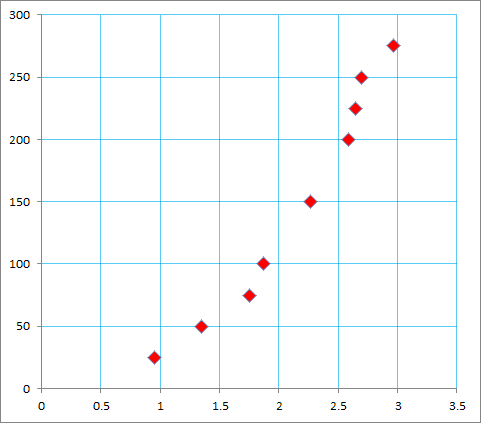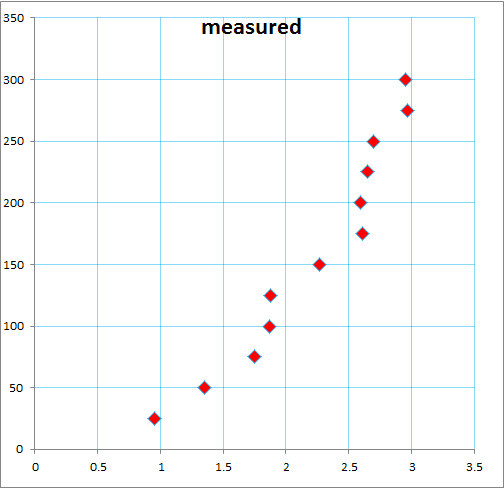# Rolling Marble + Air resistance = Displacement?

Thread moved from the technical PF forums so no Homework Help Template is shown
A marble rolls down an inclined plane with an angle of elevation of 5 degrees, roughly 4.9sin(5)m/s/s, and at normal pressure temperature NPT what would I need to add to S=1/2 at^2 to include the air resistance considering the marble is a sphere with a radius of 0.85cm and mass of 20g. The whole sphere is assumed to be in contact with the air. I know the air resistance formula however when I create the model it simply is not logical. It was 1/2at^2-(air resistance)/mass, what could be wrong with it?

## Answers and Replies

BvU
Science Advisor
Homework Helper
Hi Jon,

What about dimensions ?
I know the air resistance formula
we don'tso please post

I'm sorry I did not mean to be arrogant
The formula I used was Fdrag=-1/2CpAv^2 p=air density C=1/2 A=cross section and I have used (4.9sin(5)t)^2 for velocity squared since v=at right ? SInce initial velocity is zero.
What did you mean by dimensions? I don't know how to include anymore dimensions of a sphere other than its radius.

BvU
Science Advisor
Homework Helper
What did you mean by dimensions?
##{1\over 2} at^2## has the dimension of length. You can not add or subtract anything with a different dimension, such as force over mass (with dimension of acceleration).

Perhaps you meant to write the equation of motion $$ma = mg\sin(5^\circ) - C_d \,A \,{1\over 2} \rho v^2 \quad ?$$ but that's not so easily solved.

In your situation I would estimate an average air resistance friction force and check if it can be ignored or used as a small correction on ##mg\sin(5^\circ)##. Also check terminal velocity and compare with ##\sqrt {2g\Delta h}##.

Depending on your experiment there might also be some rolling resistance (gutter?).

And: rolling without sliding also requires some angular considerations....O.O Thx , I should look into that, I must have made some errors along the way. Yes it was a gutter but I chose to neglect the friction of that since the shape is a sphere, hopefully it shouldn't matter too much. I am trying to get my maths model to fit within 5% of experimental values, or at least provide insight as to why the error exceeds 5% if I can't find any mathematical causes. Thanks again

Orodruin
Staff Emeritus
Science Advisor
Homework Helper
Gold Member
but that's not so easily solved.
That depends on your definition of ”easily”, it is a separable differential equation.

haruspex
Science Advisor
Homework Helper
Gold Member
2020 Award
4.9sin(5)m/s/s
It is a rolling sphere, not a frictionless block.

I am "inclined" to believe that the friction of a rolling sphere can be neglected when measuring in cm for a distance of 3m, but please correct me if you can find a formula to do so. Pun intended.

Or do you mean angular momentum and stuff? If that makes a difference then I would just go back to learning theory since I cant even explain a rolling marble...

BvU
Science Advisor
Homework Helper
Could you provide some more context ? Setup, measurements (are you preparing or are you already evaluating ?), etc. Don't the measurements tell you where things start to deviate from expected behaviour ?

I am evaluating, the problem is I can't incorporate the drag equation into the model just as you said it was difficult to solve. For context, the timings were done manually with stopwatches and the plane was made out of smooth wood, slightly convex so a bit of outliers due to oscillations. For results, (x,y) refers to (time(s),displacement(cm)) : (0.95,25) (1.35,50) (1.75,75) (1.87,100) (2.27,150) ( (2.59,200) (2.65,225) (2.7,250) (2.97, 275) I have excluded some outliers but thats the raw data anyways. Thx for asking

haruspex
Science Advisor
Homework Helper
Gold Member
2020 Award
Or do you mean angular momentum and stuff?
Yes, regardless of the size and mass, the rotation will take a fixed fraction of the KE.

Oh. That makes sense. Appreciate that.

haruspex
Science Advisor
Homework Helper
Gold Member
2020 Award
I can't incorporate the drag equation into the model just as you said it was difficult to solve.
You have an equation of the form ##\dot v=A-Bv^2##. You can certainly do the first stage, getting t as a function of v. Are you familiar with partial fractions?
But the second stage, relating distance to time, looks tougher.

Not familiar with partial fractions, but I am comfortable with polynomials and hopefully that level of algebra . I ll try to get comfortable with it ASAP and maybe fit in the equation by tomorrow.

BvU
Science Advisor
Homework Helper
On the basis of what argument have you excluded your outliers ?#### Attachments

Oh its like ones that go crazy, say different displacement same time which would make the graph not even a function.

Orodruin
Staff Emeritus
Science Advisor
Homework Helper
Gold Member
Oh its like ones that go crazy, say different displacement same time which would make the graph not even a function.
That's not really a good reason to exclude outliers. The appropriate way to do it is to include errors in the measurements and do a statistical analysis.

BvU
Science Advisor
Homework Helper
I am trying to get my maths model to fit within 5% of experimental values, or at least provide insight as to why the error exceeds 5% if I can't find any mathematical causes.
maths model being ##s= {1\over 2} at^2## with a = ##g\sin(5^\circ)##, right ?

So what does your model predict for ##\ s\ ## at ##\ t=## 3 s ?

haruspex
Science Advisor
Homework Helper
Gold Member
2020 Award
Oh. That makes sense. Appreciate that.
So does that help? It should make a difference of about 15% to the top speed.
am trying to get my maths model to fit within 5% of experimental values

I went to calculate the model with air resistance, I do not know how to include KE lost to angular momentum yet. And the model gave me a concave down parabola which is not supposed to happen right? I used the formula Fnet= Fgravity-Fdrag and the drag formula I used was F=-bv^2. b was 0.7 ish for my marble and I differentiated acceleration due to gravity for the velocity, is that even correct? Anyways, Fnet turned out to be negative so any reasons why this has gone ridiculously wrong? Thanks for the outlier tip btw, (1.88, 125) (2.61, 175) (2.95, 300) here are the ones I excluded.

BvU
Science Advisor
Homework Helper
is that even correct
There are a few factors missing from your formula so no wonder the 'resistance' you calculate is a bit hefty.

BvU
Science Advisor
Homework Helper
I differentiated acceleration due to gravity for the velocity
No. It is the other way around: ##\quad \vec a = \displaystyle {d \vec v \over dt}##

BvU
Science Advisor
Homework Helper
(1.88, 125) (2.61, 175) (2.95, 300) here are the ones I excluded.So why exclude actual measurements ?